# Notes on Data Structures And Algorithms – Linked List

A linked list is a sequence of data structures, which are connected together via links.

A linked list is a sequence of nodes that contain items. Each node is connected to another node. The Linked list is the second most-used data structure after array. Following are the important terms to understand the concept of Linked List.

• Node− Each node of a linked list can store data called an element.
• Next − Each node of a linked list contains a link to the next node called Next.
• Linked List − A Linked List contains the connection link to the first node called First.

A Linked list can be visualized as a chain of nodes, where every node points to the next node.

As per the above illustration, the following are the important points to be considered.

• Linked List contains a node element called first.
• Each node carries a data field(s) and a link field called next.
• Each node is linked with its next node using its next link.
• The last node carries a link as null to mark the end of the list.

Below are the various types of linked lists,

• Simple Linked List − items are navigated in a forward direction.
• Doubly Linked List − items are navigated both in forwarding and backward direction.
• Circular Linked List − the last item contains a link to the first element as the next and the first element has a link to the last element as the previous.

## Basic Operations

Below are the basic operations supported by a list,

• Insertion − Adds an element at the beginning of the linked list.
• Deletion − Deletes an element at the beginning of the linked list.
• Display −  The complete linked list is displayed.
• Search −  An element is searched using the given key.
• Delete − An element is deleted using the given key.

## Insertion Operation

Adding a new node in a linked list is a single-step activity. We shall learn this with diagrams as shown below. First, create a node using the same structure and find the location where it has to be inserted.Let’s say that we are inserting a node B (NewNode), between A (LeftNode) and C (RightNode). Then point B.next to C −

```NewNode.next −> RightNode;
```

It looks like this, interesting na!!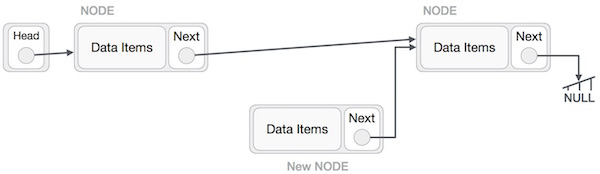Now, the next node at the left should point to the new node.

`LeftNode.next −> NewNode;`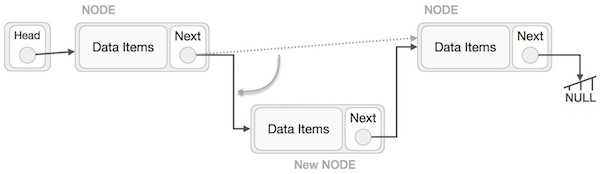This will put the new node in the middle of the two. The new list must look like this,A similar process must be followed if the node is being inserted at the beginning of the list. While inserting it at the end, the second last node of the list should point to the new node and the new node will point to NULL.

## Deletion Operation

Deletion is also a more than one-step process. We shall learn with pictorial representation. First, locate the target node to be removed, by using searching algorithms.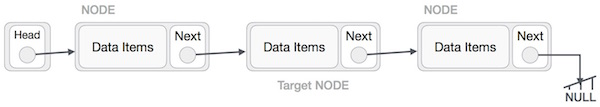The left (previous) node of the target node must point to the next node of the target node −

`LeftNode.next −> TargetNode.next;`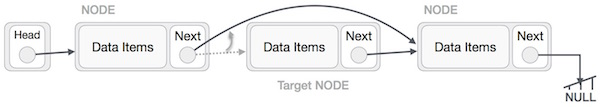This will remove the link that was pointing to the target node. Now, using the following code, we will remove what the target node is pointing at.

`TargetNode.next −> NULL;`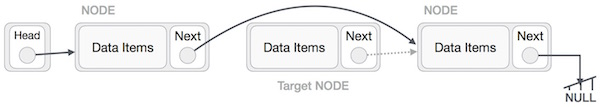We need to use the deleted node. It’s better to simply deallocate memory and wipe off the target node completely.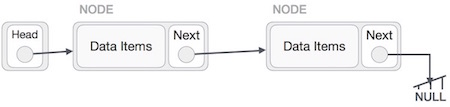## Reverse Operation

This operation is a meticulous one. We need to make the last node to be pointed by the head node and reverse the whole linked list.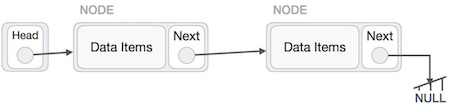Initially, we traverse to the end of the list. It should be pointing to NULL. Now, we shall make it point to its previous node −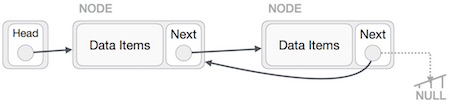We have to make sure that the last node is not the last node. So we’ll have a temp node, which looks like the head node pointing to the last node. Now, we shall make all left side nodes point to their previous nodes one by one.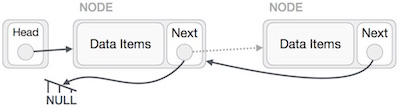Except for the node (first node) pointed by the head node, all nodes should point to their predecessor, making them their new successor. The first node will point to NULL.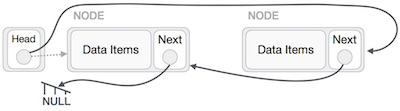We’ll make the head node point to the new first node by using the temp node.The linked list is now reversed.

Please find Codes of 1D Arrays, 2D Arrays, Strings, Pointers, Data Structures, Files, Linked lists, and MISC on the below page:

Top 100+ C Programming codes – KLE Technological University